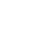×Want to shop in US Dollar? Try our Webshop specifically for North America!

Rammstein Sneaker ”Logo Allover”

••40.00 EUR
In stock.

Black sneaker with grey Rammstein logos allover. Black laces and Rammstein lettering on the heel.

Please do NOT use the box as a shipping box for returns.

UK - EU - US (women) - US (men)

4 = 36 = 6.5 = 5
4.5 = 37 = 7 = 6
5 = 38 = 7.5 = 6
6 = 39 = 8.5 = 7
6.5 = 40 = 9 = 7.5
7.5 = 41 = 10 = 8.5
8 = 42 = 10.5 = 9
9 = 43 = 11.5 = 10
9.5 = 44 = 12 = 10.5
10.5 = 45 = 13 = 11.5
11 = 46 = 13.5 = 12
11.5 = 47 = 14 = 13